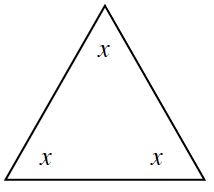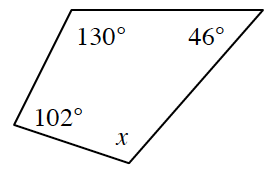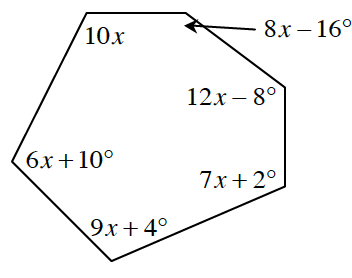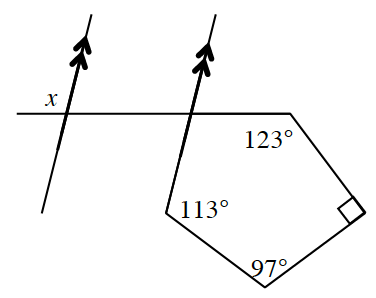### Home > CCG > Chapter Ch8 > Lesson 8.1.5 > Problem8-60

8-60.

Solve for $x$ in each diagram below.

Review the Math Notes box in Lesson 8.1.5 for help determining the sum of the interior angles of a polygon.

1.$x = 60º$

1.$x = 82º$

1.$10x + (8x - 16) + (12x - 8) + \text{... ?}$

1.How are $\angle x$ and the missing angle in the polygon related?